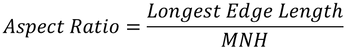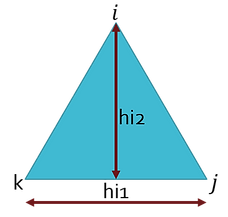top of page

## How different simulation softwares calculate Aspect ratio for 2D Elements

This article explains how different finite element solvers calculates Aspect Ratio. Since, Each solver has their own method of calculating Element Quality Parameters it is always better to understand how these checks are done before proceeding with the solver. We have considered the following five different solvers for explaining the Aspect Ratio Calculation. In our previous Article on "Aspect Ratio Calculation for 2D Elements" we have already explained how "Ansys solver" calculates Aspect Ratio, so we are not mentioning it again here.

##### 1. Hypermesh:
###### 1.1 Aspect Ratio Calculation for Triangular Element:

Aspect ratio: The ratio between longest edge of an element to either its shortest edge or the shortest distance from a corner node to the opposing edge is called Aspect Ratio.Minimum Length: Hypermesh uses the following three methods to calculate minimum length.

1. The shortest edge (in which the length of the shortest edge of each element is used).

2. The minimal normalized height (which is more accurate, but more complex).

3. The minimal height (which is the same as minimal normalized height but without a scaling factor).

Minimal Normalized Height (MNH):

• Find the perpendicular distance from each node to its opposite side (hi, hj & hk).

• Hence, the MNH is minimum of (hi, hj & hk) multiplied by scaling factor [2/√3] (scaling factor ensures that for Equilateral triangle MNH is same as the minimum side length).Aspect Ration for Equilateral Triangle:

Assume Each Side of triangle: ”a”

Each Corner Angle: 60 degree

Find out the perpendicular distance between each node to its opposite side and find the MNH with scaling factor.###### 1.2 Aspect Ratio Calculation for Quadrilateral Element:

Aspect ratio: The ratio between longest edge of an element to either its shortest edge or the shortest distance from a corner node to the opposing edge is called Aspect Ratio.Minimum Length: Hypermesh uses the following methods to calculate minimum length.

1. The shortest edge (in which the length of the shortest edge of each element is used).

2. The minimal normalized height (which is more accurate, but more complex).

Minimal normalized height (MNH):

• For each corner node, draw perpendicular line to the other two sides which are not containing that node.

• With respect to the figure, from node “j” draw perpendicular lines to sides “lj & lk” as shown. Measure the lengths “hj1 & hj2”.

• Similarly, Repeat the above procedure for all other nodes (I, k & l) and find out their lengths (total 8 Lengths).

• Thus, Minimal normalized height (MNH) is taken to be the minimum of all eight lines and the four edge lengths (thus, the minimum of 12 possible lengths).Example: Aspect Ratio of a rectangle:

• MNH = Min.Edge length=5

• Aspect Ratio=10/5 = 2##### 2. MSC Patran:
###### 2.1 Aspect Ratio Calculation for Triangular Element:

Aspect Ratio: The Maximum ratio of the Length of a side to Perpendicular distance from that side to its opposite node, then multiplied by √3/2 is the aspect ratio for Triangles.• √3/2  is a factor based on Equilateral triangle.

• The process is performed for each of the three sides, and the largest value of the three is reported as the Aspect Ratio.

###### 2.2 Aspect Ratio for Quadrilateral Element:

It is the maximum of the two length ratios rectangles formed with L1 & L2.• Join mid points of opposite sides (L1 & L2).

• Fixing L1 as axis, draw parallel lines to L1 at mid points of the other opposite sides at E & G.

• Draw perpendicular lines to L1 at F & H.

• Find the Ratio between the Max. Side to Mini. side of the rectangle formed.

• Similarly, Fixing L2 as axis, draw parallel lines to L2 at mid points of the other opposite sides at F & H.

• Draw perpendicular lines to L2 at E & G.

• Find the ratio between the Max. side to Mini. Side of the rectangle formed.

So, The Aspect Ratio is Maximum of the two length ratios formed by L1 & L2.

##### 3. NX Nastran:
###### 3.1 Aspect Ratio Calculation for Triangular & Quadrilateral Elements:

Aspect ratio is defined as the ratio between longest element edge to shortest element edge.

As per NX Nastran, Very high aspect ratio should also be avoided, although it is no longer true that accuracy degrades rapidly with aspect ratios as it once did with some of  he obsolete elements.Aspect Ratio is calculated for CQUAD4, CQUAD8, and CTRIA6 elements using above method.

##### 4. I-DEAS:
###### 4.1 Stretch/Aspect Ratio Calculation for Triangular Elements:

The radius of the largest circle that fits within the element is divided by the longest edge, then multiplied by the square root of 12 is called stretch ratio. Inverse of Stretch is called Aspect Ratio.Note: √(12 )  is the factor for Equilateral triangle and r=a/√12

###### 4.2 Stretch/Aspect Ratio Calculation for Quadrilateral Elements:

The minimum edge length is divided by the maximum diagonal length. The result is multiplied by square root 2.Note: √2  is the factor for Square and dmax=a√2

##### 5. Abaqus:
###### 5.1 Aspect Ratio Calculation for Triangular and Quadrilateral Elements:

Aspect ratio for Tria. And Quad. Elements: The ratio between longest edge of an element to its shortest edge is called Aspect Ratio.References:

1. Hypermesh user's guide**.

2. Abaqus/cae user’s guide**.

3. MSC Patran reference manual**.

4. MSC Nastran Getting Started User’s Guide**.

5. NX Nastran User's Guide**.

Note (**): Ansys, Hypermesh, Abaqus, MSC Patran, NX Nastran are Registered trademarks of their respective owners.

Hypermesh
MSC Patran
NX Nastran
I-DEAS
Abaqus

bottom of page